Overview: SURVEYPHREG Procedure

The SURVEYPHREG procedure performs regression analysis based on the Cox proportional hazards model for sample survey data. Cox’s semiparametric model is widely used in the analysis of survival data to estimate hazard rates when adequate explanatory variables are available. The procedure provides design-based variance estimates, confidence intervals, and hypothesis tests concerning the parameters and model effects. See Chapter 3: Introduction to Statistical Modeling with SAS/STAT Software, and Chapter 14: Introduction to Survey Sampling and Analysis Procedures, for an introduction to the basic concepts of survey data analysis; see Chapter 13: Introduction to Survival Analysis Procedures, for an introduction to the basic concepts of survival analysis.

The survival time of each member of a finite population is assumed to follow its own hazard function,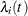, expressed as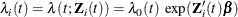where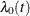is an arbitrary and unspecified baseline hazard function,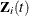is the vector of explanatory variables for the ith population unit at time t, andis the vector of unknown regression parameters.

The finite population regression parameter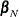is defined as the maximizer of the partial log likelihood when the entire finite population is observed. The SURVEYPHREG procedure produces a sample-based estimate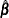of the proportional hazards regression parametersfor the finite population by maximizing the partial pseudo-log-likelihood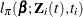based on observed covariatesand observed survival time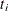. The procedure also produces an estimate of the sampling variance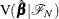, which assumes that the values of the finite population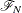are fixed. For statistical inference, PROC SURVEYPHREG incorporates complex survey sample designs, including designs with stratification, clustering, and unequal weighting.

The procedure also allows time-dependent explanatory variables. An explanatory variable is time-dependent if its value for any given individual can change over time. Time-dependent variables have many useful applications in survival analysis. You can include time-dependent variables such as blood pressure or blood chemistry measures that vary with time during the course of a study. You can also use time-dependent variables to test the validity of the proportional hazards model.

Several optimization techniques are available in SURVEYPHREG to maximize the log likelihood. Hazard ratio estimates can also be obtained along with parameter estimates. Sampling errors of the regression parameters and hazard ratios are computed by using either the Taylor series (linearization) method or one of the replication (resampling) methods that are based on complex sample designs (Binder, 1983; Wolter, 2007; Särndal, Swensson, and Wretman, 1992; Binder, 1992; Lohr, 2010; Fuller, 2009). These variance estimators essentially assume the finite population as fixed and estimate the variability due to the random sample selection mechanism.

The remaining sections of this chapter contain information about how to use PROC SURVEYPHREG, information about the underlying statistical methodology, and some applications of the procedure. The section Getting Started: SURVEYPHREG Procedure introduces PROC SURVEYPHREG with an example. The section Syntax: SURVEYPHREG Procedure describes the syntax of the procedure. The section Details: SURVEYPHREG Procedure summarizes the statistical techniques employed in PROC SURVEYPHREG. The section Examples: SURVEYPHREG Procedure includes some additional examples of useful applications. Experienced SAS/STAT software users might decide to proceed to the Syntax section, while other users might choose to read both the Getting Started and Examples sections before proceeding to Syntax and Details.